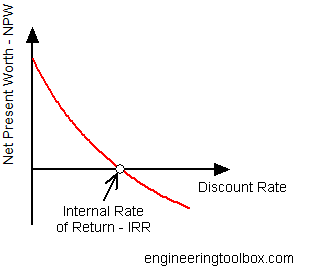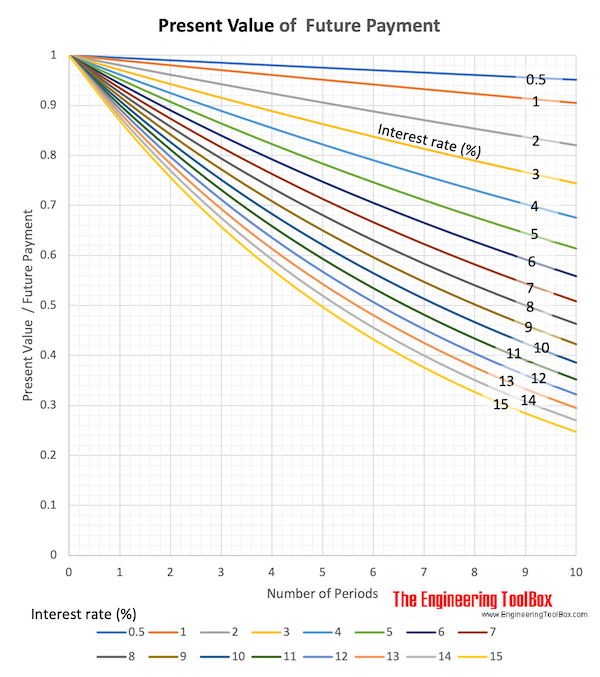Engineering ToolBox - Resources, Tools and Basic Information for Engineering and Design of Technical Applications!

# Internal Rate of Return - IRR

## Internal Rate of Return (IRR) - the break-even interest rate.

A primary measure of an investments worth (or value) is based on yield and known as the internal rate of return - IRR.

The internal rate of return can be defined as the break-even interest rate which equals the Net Present Worth - NPW - (Net Present Value) of a project in and out cash flows.

P(irr) = Fcash_in - Fcash_out

= 0                    (1)

where

P = Present Worth (Value)

irr = internal rate of return

Fcash_in = future cash flow in (discounted)

Fcash_out = future cash flow out (discounted)

(1) can be expressed as

P(irr) = F0 / (1 + irr)0 + F1 / (1 + irr)1 + F2 /(1 + irr)2 + .... + Fn /(1 + irr)n

= 0                    (2)

where

F0..n = cash flow in period 0 to n (positive value for cash flow in - negative value for cash flow out)

For a given cash flow equation (2) can be solved by iteration.### Cash Flow Internal Rate of Return Calculator

• money out - negative values
• money in - positive values

Cash Flows - Period

0

1

2

3

4

5

6

7

8

9

10

11

12

13

14

15

16

17

18

19

20### Minimum Attractive Rate of Return - MARR

Minimum Attractive Rate of Return - MARR - represents the required or minimum acceptable Internal Rate of Return for a project investment.

## Related Topics

• Economics - Engineering economics - cash flow diagrams, present value, discount rates, internal rates of return - IRR, income taxes, inflation.

## Engineering ToolBox - SketchUp Extension - Online 3D modeling!

Add standard and customized parametric components - like flange beams, lumbers, piping, stairs and more - to your Sketchup model with the Engineering ToolBox - SketchUp Extension - enabled for use with the amazing, fun and free SketchUp Make and SketchUp Pro .Add the Engineering ToolBox extension to your SketchUp from the SketchUp Pro Sketchup Extension Warehouse!

Translate

## Privacy

We don't collect information from our users. Only emails and answers are saved in our archive. Cookies are only used in the browser to improve user experience.

Some of our calculators and applications let you save application data to your local computer. These applications will - due to browser restrictions - send data between your browser and our server. We don't save this data.

## Citation

• Engineering ToolBox, (2008). Internal Rate of Return - IRR. [online] Available at: https://www.engineeringtoolbox.com/internal-rate-of-return-irr-d_1235.html [Accessed Day Mo. Year].

Modify access date.

. .

#### Scientific Online Calculator6 24